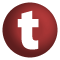# Ordered

#### trait Ordered[A] extends Comparable[A]

A trait for totally ordered data.

Note that since version 2006-07-24 this trait is no longer covariant in a.

It is important that the equals method for an instance of Ordered[A] be consistent with the compare method. However, due to limitations inherent in the type erasure semantics, there is no reasonable way to provide a default implementation of equality for instances of Ordered[A]. Therefore, if you need to be able to use equality on an instance of Ordered[A] you must provide it yourself either when inheiriting or instantiating.

It is important that the hashCode method for an instance of Ordered[A] be consistent with the compare method. However, it is not possible to provide a sensible default implementation. Therefore, if you need to be able compute the hash of an instance of Ordered[A] you must provide it yourself either when inheiriting or instantiating.

known subclasses: Value, StringLike, Value, Value, Value, Value, Value, Value, Value, Value, Value, Value, Value, Value, Value, Value, Value, Value, Value, Value, Value, RichByte, RichFloat, RichBoolean, RichChar, RichShort, RichLong, RichInt, RichDouble

1. Comparable
2. AnyRef
3. Any

### Value Members

5. #### def compare(that: A): Int

Result of comparing `this` with operand `that`

Result of comparing `this` with operand `that`. returns `x` where `x < 0` iff `this < that` `x == 0` iff `this == that` `x > 0` iff `this > that`

attributes: abstract

7. #### def equals(arg0: Any): Boolean

This method is used to compare the receiver object (`this`) with the argument object (`arg0`) for equivalence

This method is used to compare the receiver object (`this`) with the argument object (`arg0`) for equivalence.

The default implementations of this method is an equivalence relation:

• It is reflexive: for any instance `x` of type `Any`, `x.equals(x)` should return `true`.
• It is symmetric: for any instances `x` and `y` of type `Any`, `x.equals(y)` should return `true` if and only if `y.equals(x)` returns `true`.
• It is transitive: for any instances `x`, `y`, and `z` of type `AnyRef` if `x.equals(y)` returns `true` and `y.equals(z)` returns `true`, then `x.equals(z)` should return `true`.

If you override this method, you should verify that your implementation remains an equivalence relation. Additionally, when overriding this method it is often necessary to override `hashCode` to ensure that objects that are "equal" (`o1.equals(o2)` returns `true`) hash to the same `Int` (`o1.hashCode.equals(o2.hashCode)`).

arg0

the object to compare against this object for equality.

returns

`true` if the receiver object is equivalent to the argument; `false` otherwise.

definition classes: AnyRef ⇐ Any
8. #### def hashCode(): Int

Returns a hash code value for the object

Returns a hash code value for the object.

The default hashing algorithm is platform dependent.

Note that it is allowed for two objects to have identical hash codes (`o1.hashCode.equals(o2.hashCode)`) yet not be equal (`o1.equals(o2)` returns `false`). A degenerate implementation could always return `0`. However, it is required that if two objects are equal (`o1.equals(o2)` returns `true`) that they have identical hash codes (`o1.hashCode.equals(o2.hashCode)`). Therefore, when overriding this method, be sure to verify that the behavior is consistent with the `equals` method.

definition classes: AnyRef ⇐ Any
9. #### def toString(): String

Returns a string representation of the object

Returns a string representation of the object.

The default representation is platform dependent.

definition classes: AnyRef ⇐ Any